# Lattice Vibrations

## Lattice vibrations

The oscillations of atoms in a solid about their equilibrium positions. In a crystal, these positions form a regular lattice. Because the atoms are bound not to their average positions but to the neighboring atoms, vibrations of neighbors are not independent of each other. In a regular lattice with harmonic forces between atoms, the normal modes of vibrations are lattice waves. These are progressive waves, and at low frequencies they are the elastic waves in the corresponding anisotropic continuum. The spectrum of lattice waves ranges from these low frequencies to frequencies of the order of 1013 Hz, and sometimes even higher. The wavelengths at these highest frequencies are of the order of interatomic spacings. See Crystal structure, Vibration, Wave motion

At room temperature and above, most of the thermal energy resides in the waves of highest frequency. Because of the short wavelength, the motion of neighboring atoms is essentially uncorrelated, so that for many purposes the vibrations can be regarded as those of independently vibrating atoms, each moving about its average position in three dimensions with average vibrational energy of 3kT, where k is the Boltzmann constant and T the absolute temperature. The wave character of the vibrations is needed, however, to describe heat transport by lattice waves. Also, lattice vibrations interact with free electrons in a conducting solid and give rise to electrical resistance. The temperature variation at low temperatures provides evidence that this interaction is with waves. See Electrical resistivity

Scattering of lattice waves by defects increases with increasing frequency (f); its variation depends on the nature of the defect. Scattering by external and internal boundaries is almost independent of frequency, thus dominating at low frequencies and hence at low temperatures. A study of the thermal conductivity of nonmetallic crystals as function of temperature yields information about the defects present, and about the anharmonic nature of the interatomic forces in the crystal lattice. See Crystal defects, Thermal conduction in solids

## Lattice Vibrations

one of the main types of internal motion of a solid, in which the constituent particles (atoms or molecules) oscillate about equilibrium positions—the lattice points. For example, lattice vibrations in the form of standing or traveling sound waves arise whenever a crystal is acted upon by an external force that varies over time. However, even in the absence of external influences a steady state of vibrations is established in a crystal that is in thermal equilibrium with its environment, just as a fixed distribution of atoms or molecules is established in a gas according to their rate of translatory motion.

The nature of lattice vibrations depends on the symmetry of the crystal, the number of atoms in its unit cell, the type of chemical bond, and the type and concentration of the crystal defects. The higher the temperature, the greater will be the displacement u of atoms in the process of oscillation, but even up to the melting point, at which a solid becomes a liquid, it is much smaller than the lattice constant. The forces that tend to hold the atoms in equilibrium positions are proportional to their relative displacements as if they were interconnected to each other by springs (Figure 1). The representation of a crystal as a set of particles connected by ideally elastic forces is called a harmonic approximation.

In a crystal consisting of N unit cells of n atoms each there exist 3nN — 6 types of the simplest vibrations in the form of standing waves, which are called normal (or natural) oscillations, or modes. Their number is equal to the number of degrees of freedom of the set of particles in the crystal, less the three degrees of freedom that correspond to the translatory motion and the three that correspond to the rotary motion of the crystal as a whole. The number 6 may be disregarded, since 3nN is a quantity of the order of 1022-1023 per cubic centimeter of the crystal.

In the course of normal vibration all particles of the crystal oscillate about their own equilibrium positions with the same constant frequency ω, like simple harmonic oscillators. All possible normal vibrations may be present simultaneously in a crystal, and each occurs as if the others were not present. Any motion of atoms in a crystal that does not disrupt its microstructure may be represented as a superposition of the normal vibrations of the crystal.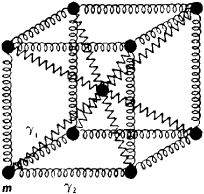Figure 1. Representation of a body-centered cubic crystal in the form of a set of particles of mass m that are interconnected by springs with stiffnesses r1, and r2

Every standing wave of a normal vibration may in turn be represented as two elastic plane traveling waves that are propagating in opposite directions (normal waves). In addition to the frequency ω, a plane traveling wave is characterized by the wave vector k, which defines the direction of motion of the wave front and the wavelength λ = 2π/k, and also by the polarization, which determines the nature of the individual particle motion. In the general case elliptical polarization takes place. Here each atom describes an ellipse about its own equilibrium position (Figure 2), and the normal to the plane of the ellipse does not coincide in direction with k. The elliptical orbits are identical for identical atoms that occupy equivalent positions in the lattice. In crystals where every point is a center of symmetry, all normal waves are plane-polarized: the atoms in any normal vibration perform reciprocating motions about their equilibrium positions.Figure 2. Elliptical polarization of elastic waves in a crystal; k is a wave vector

Dispersion of normal waves. For every value of k there exist 3n of normal waves with different polarization. They are numerated by the integral variable σ = 1, 2, . . . , 3n and are called branches of normal vibrations. For waves of a given type of σ the quantities ω and k cannot be arbitrary but are interconnected by a specific relationship ω = ω(k,σ), called the dispersion law. For example, if a crystal is represented in the form of a set of identical atoms of mass m that lie at equal distances a from each other and are joined in pairs by springs having stiffness γ in such a way that they form an infinite chain and can be displaced only along its axis (Figure 3,a), the unit cell consists of one particle and one branch of the normal vibration frequency exists, with the dispersion law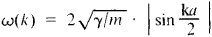In a diatomic linear chain (Figure 3,b) the cell contains two particles with masses m and M and has two branches with a more intricate dispersion law (Figure 4).

The elastic waves in a crystal always have dispersion. In particular, their phase velocity usually differs from the group velocity at which the vibratory energy is transmitted through the crystal. Although the frequency ω of elastic waves propagated in a continuous medium increases indefinitely with an increase in k, some maximum vibration frequency ωmax, usually about 1013 hertz (Hz), exists in a crystal because of the periodic location of the atoms and the finite magnitude of the force binding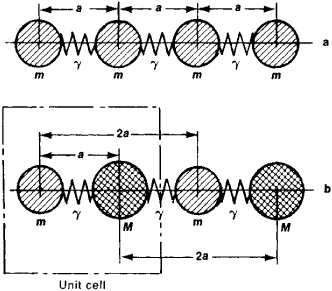Figure 3. The simplest models of a crystal: (a) linear monatomic chain, (b) linear diatomic chain; (m) and (M) masses of the two particles that make up a unit cell

them. Natural frequencies may not entirely fill the interval between ω = 0 and ω = ωmax and may contain vacant areas (forbidden bands) that separate two successive branches (Figure 4). There is no forbidden band between adjacent branches if the branches overlap. Vibrations that correspond to the forbidden band or have frequency ω > ωmax cannot propagate in a crystal and attenuate rapidly.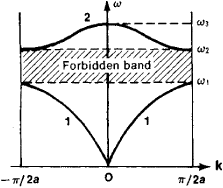Figure 4.

Note to Figure 4. Dispersion law of a diatomic linear chain: (1) acoustical branch, (2) optical branch,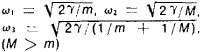Acoustical and optical branches. The first three branches of vibrations, with σ = 1, 2, 3, are called acoustical branches. If the wavelength λ greatly exceeds the longest period of a space lattice (that is, k is small), the branches are characterized by the linear dispersion law ω = c.k. These are ordinary sound waves, and c is the phase velocity of their propagation, which depends on the direction of propagation and on polarization. They are plane-polarized in one of the three mutually perpendicular directions according to the three values of σ = 1, 2, 3, and correspond to vibrations of the crystal as a continuous medium. In an anisotropic crystal it usually is the case that none of these directions coincides with the direction of wave propagation, that is, with k. Only in an elastic isotropic medium do sound waves have purely longitudinal and purely cross polarization. The acoustical branches cover the band from zero to about 1013 Hz. However, as the wavelength decreases the dispersion law becomes more complex.

For the other 3(n — 1) branches of the frequency, the displacements of the atoms in the course of the vibrations corresponding to a long wavelength take place in such a way that the center of mass of an individual unit cell is at rest. In ionic crystals, whose unit cell consists of ions of opposite sign, motion of this type can be initiated by an alternating electric field, such as a light wave, usually one whose frequency lies in the infrared region. Therefore, these branches are said to be optical. The acoustical branch was named after the initial zone (Figure 4), since the initial zone of the acoustical branch is ordinary sound.

Phonons. A set of moving quasiparticles with momentum p = hk and energy ξ = , where h is Planck’s constant, may be placed in correspondence with every traveling plane wave with vector k and frequency ω. These quasiparticles are the field quanta of crystal lattice vibrations and are called phonons by analogy with photons, the quanta of the electromagnetic field.

Influence of Lattice Vibrations on Crystal Properties. The higher the temperature of a crystal, the more intensively its atoms oscillate about their equilibrium position. When the amplitude of the vibrations exceeds some critical value, melting begins and the crystal structure is destroyed. As the temperature drops, the amplitude decreases, and it reaches a minimum when T = 0°K. According to the laws of quantum mechanics it is impossible for atoms to stop completely and for their energy to become zero; when T = 0°K they perform zero-point oscillations. Since the energy of zero-point oscillations is usually insufficient to cause melting of solid, all liquids eventually solidify as the temperature decreases. The only exception is helium, which remains a liquid all the way down to 0°K and solidifies only under pressure.

The lattice heat capacity is a quantitative characteristic of a crystal’s ability to store heat in the form of oscillatory energy. The heat capacity per atom is found to be approximately equal to 3kB, where kB is the Boltzmann constant, at high temperatures (Dulong and Petit’s law) and proportional to T3 when T approaches 0°K.

In metals and semiconductors there are —in addition to atoms or ions—free electrons, which create an electric current in the presence of an electric field. The laws governing their motion are such that they pass unhindered through an ideal crystal consisting of atoms in a state of zero-point oscillations. Therefore, resistance of an electric current when T -> 0°K arises only to the extent that crystals always contain defects that scatter electrons. However, at temperatures T > 0°K the oscillations randomly disrupt the ideally periodic nature of the lattice and create supplementary electric resistance, called lattice, or phonon, electric resistance. Upon collision with oscillating atoms, electrons transmit to the crystal skeleton part of the energy of their own directed translatory motion, which is released as Joule heat.

Anharmonicity. In reality restoring forces are not strictly proportional to the displacements of atoms from equilibrium positions, and the vibrations of a crystal are not strictly harmonic (anharmonicity). The nonlinearity of interatomic forces is small, since the amplitudes of the oscillations are small. However, by virtue of this nonlinearity individual normal vibrations are not independent but rather are interconnected, and resonance between them is possible, as in a system of coupled pendulums.

Anharmonicity plays the same role in the process of establishing thermodynamic equilibrium in crystals as do the collisions of particles in a gas. In particular, this explains the thermal expansion of crystals, the deviation from Dulong and Petit’s law in the high-temperature region, and the difference between the isothermic and adiabatic elastic constants of a solid and their dependence on temperature and pressure.

When a solid is heated nonuniformly, heat fluxes arise in it. In metals most of this heat is transferred by electrons; in dielectrics, by normal waves (phonons). Therefore if we have in mind dielectrics or the lattice part of the thermal conductivity of metals, in the absence of anharmonicity the heat flux would propagate with the velocity of normal waves—that is, at approximately the speed of sound. Because of anharmonicity the waves in a heat flux exchange energy and interfere with each other. In the process of interference of loss of the total momentum of the heat flux takes place. As a result, thermal resistance arises, and the thermal energy is transferred at the diffusion rate, which is much slower than the rate or propagation of elastic energy such as a sound wave. Anharmonicity is also one reason for the attenuation of ultrasound in crystals.

Local and quasi-local vibrations. The nature of lattice vibrations is significantly affected by defects in the crystal lattice. The stiffness of interatomic bonds and the masses of particles in the region of a defect differ from those of an ideal crystal, which is called the standard or the matrix. As a result, the normal waves are not plane. For example, if a defect is an impurity atom of mass m0, coupled with its neighbors by “springs” of stiffness γ0, its natural frequency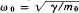may fall within the forbidden band of matrix frequencies. Only the impurity atom takes an active part in such vibration; therefore, it is called a local vibration. Since there are always many defects in a real crystal, local vibration, having been excited at one defect, may extend to another, as in the resonance of identical, weakly coupled pendulums. Therefore, local vibrations have an entire spectrum of frequencies that form the impurity zone of the lattice vibration frequencies.

In addition to local vibrations, so-called quasi-local vibrations may exist in the low-frequency region. In particular, such vibrations can be found in a crystal with heavy impurity atoms. At low temperatures quasi-local vibrations sharply increase the lattice heat capacity, the coefficient of thermal expansion, and thermal and electric resistance. For example, 2–3 percent impurity atoms that are 10 times heavier than the matrix atoms is sufficient at low T to double the lattice heat capacity and coefficient of thermal expansion.

Local vibrations of extended defects, such as dislocations, propagate along the defects in the form of waves but do not penetrate into the matrix, as in the case of point defects. The frequency of these vibrations may fall within either the forbidden or permitted bands of matrix frequencies, differing from them by their dispersion law. Such, for example, are the surface sound waves that occur near the flat boundary of a solid (Rayleigh waves).

There are various experimental methods for studying lattice vibrations. Excitation by means of infrared radiation is one method of studying local and quasi-local vibrations. It is accompanied by a resonance decrease in the transparency of the crystal and makes possible not only the detection of the vibrations but also the determination of their frequencies.

Studies of inelastic scattering of neutrons in crystals make possible determination of the dispersion law and polarization of normal vibrations. The dispersion law may also be reconstructed by using the diffusion scattering of X rays. The Mössbauer effect makes possible direct determination of the mean square displacements and momenta of atoms in the process of lattice vibrations.

### REFERENCES

Ziman, J. Elektrony ifonony. Moscow, 1962. (Translated from English.)
Ziman, J. Printsipy teorii tverdogo tela. Moscow, 1966. (Translated from English.)
Leibfried, G. Mikroskopicheskaia teoriia mekhanicheskikh i teplovykh svoistv kristallov. Moscow, 1963. (Translated from English.)
Maradudin, A. Defekty i kolebatel’nyi spektr kristallov. Moscow, 1968. (Translated from English.)
Landau, L. D., and E. M. Lifshits. Statisticheskaia fizika, 2nd ed. Moscow, 1964.
Landau, L. D., and E. M. Lifshits. Teoriia uprugosti, 3rd ed. Moscow, 1965. (Teoreticheskaia fizika, vol. 7.)
Kittel, C. Vvedenie v fiziku tverdogo tela. Moscow, 1963. (Translated from English.)

IA. A. IOSILEVSKII

References in periodicals archive ?
In the crystalline phases the bonding energy and thus the thermal conductivity is enhanced compared to the amorphous regions [1, 2], According to the theory of heat conduction, in electrically insulating materials heat is transported via lattice vibrations due to the lack of free electrons.
Hong added, This insight into how lattice vibrations can control phase stability in transition-metal oxides is needed to improve the performance of many multifunctional materials, including colossal magnetoresistors, superconductors and ferroelectrics.
Pressure and lattice vibrations alone can be viewed as controlling the reactions with protons readily available from the hexagonal plane.
The problems are arranged in topical chapters such as the Sommerfeld theory of metals, bonding in solids, normal modes of lattice vibrations, fundamentals of semiconductors, and optical properties of solids.
Each chapter presents about 15-20 problems within a text explaining a particular topic, among them inter-atomic forces and lattice vibrations, density of states, thermodynamics of non-interacting quasi-particles, and interactions and phase transitions.
The TPIspectra1000 also has a high sensitivity for the detection of crystalline lattice vibrations, which is useful for pharmaceutical polymorphic screening.
In copper oxide superconductors, lattice vibrations alone are not strong enough to maintain the necessary electron pairing at elevated temperatures.
Phonons are quantized lattice vibrations that excite the carbon atoms as they pass through.
We will use these new methods to calculate the transfer of energy from electronic states to the lattice vibrations in photoexcited Gs and to follow the redistribution of vibrational energy through to its ultimate thermalisation in the lattice.
It cannot have recourse to lattice vibrations as a degree of freedom.
They quickly realized that in these materials, lattice vibrations alone aren't strong enough to maintain such pairing at the high superconducting transition temperatures observed in the copper oxides.

Site: Follow: Share:
Open / Close